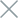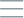• Home
• Tags
• Series
•# Binary Search Algorithm in Java

The Binary Search Algorithm is an efficient method in Java programming, used to find a specific value within a sorted array. This approach continuously divides the array into two parts and compares the search value with the middle element.

## How the Binary Search Algorithm Works

The Binary Search Algorithm begins by comparing the search value with the middle element of the array. If the search value is equal to the middle element, the algorithm returns the position of that element. If the search value is less than the middle element, the algorithm continues the search in the left half of the array. If the search value is greater, the algorithm continues the search in the right half of the array. This process repeats until the search value is found or there are no more elements to search.

• High Efficiency: This algorithm eliminates half of the elements in each step, optimizing the search for large arrays.
• Low Time Complexity: The time complexity of this algorithm is O(log n), making it effective for large datasets.

• Sorted Array Requirement: The algorithm only works with sorted arrays.

## Example and Explanation

Consider an example of using the Binary Search Algorithm to find a specific integer in a sorted integer array in Java.

``````public class BinarySearchExample {
public static int binarySearch(int[] array, int target) {
int left = 0;
int right = array.length - 1;

while (left <= right) {
int mid = left + (right - left) / 2;

if (array[mid] == target) {
return mid; // Return position if found
} else if (array[mid] < target) {
left = mid + 1;
} else {
right = mid - 1;
}
}
}

public static void main(String[] args) {
int[] numbers = { 1, 3, 5, 7, 9, 11, 13, 15 };
int target = 9;

int position = binarySearch(numbers, target);

if (position != -1) {
System.out.println("Element " + target + " found at position " + position);
} else {